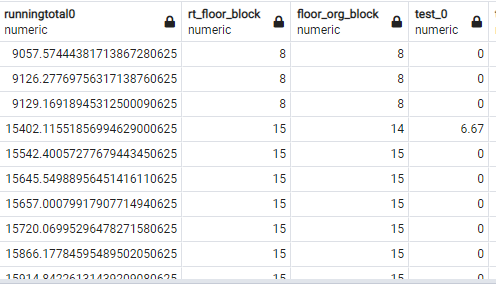# Need help with SQL query

Hi everyone,
I am working on to find the the difference in rt_floor_block 8 to 15. can someone help me with finding the difference and then add 6.67 in test_0 for each step.
8 = 6.67
9 = 6.67
10 =6.67
11 = 6.67
12 = 6.67
13 = 6.67
14 = 6.67
15 = 6.67
in some cases its jumps from 25 to 33 or 42 to 50 .

case when rt1.runningtotal0 > 0 then case when floor((rt1.runningtotal0/1024)) > (floor( ( (rt1.runningtotal0 - (rt1.orgmb))/1024) ) ) then 6.67 else 0 end end test_0

current outputTo do what you are describing, you need to be able to order the rows in the table. Rows in a table are an unordered set, even though it may look ordered when you query the table. So if you don't have a way to order, SQL Server cannot know which row comes first, and which row follows that and so on.

If you have a column, for example, ID, which can be used for ordering, you would do something like this:

``````SELECT
id,
rt_floor_block,
rt_floor_block-LAG(rt_floor_block,1,0) OVER (ORDER BY id)
FROM
tbl;
``````